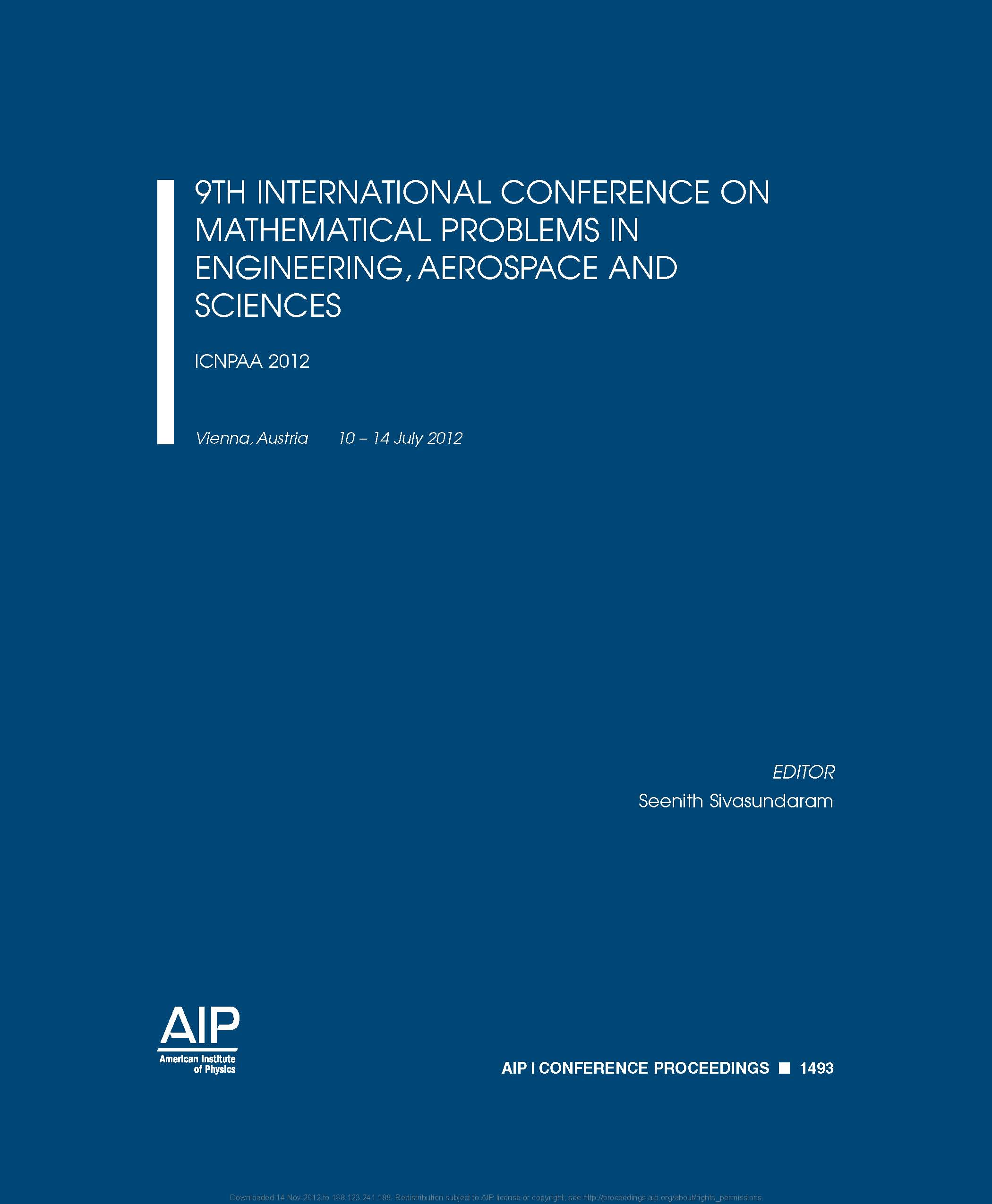• A
• A
• A
• АБB
• АБB
• АБB
• А
• А
• А
• А
• А
Обычная версия сайта

## Guaranteed Control of Feedback Linearizable Nonlinear Object

P. 13-20.

An optimal control problem is formulated for a class of nonlinear systems for which there exists a coordinate representation transforming the original system into a system with a linear main part and a nonlinear feedback. In this case the coordinate transformation significantly changes the form of original quadratic functional. The penalty matrices become dependent on the system state. The linearity of the transformed system structure and the quadratic functional make it possible to pass over from the Hamilton–Jacoby–Bellman equation (HJB) to the state dependent Riccati equation (SDRE) upon the control synthesis. Note that it is rather difficult to solve the obtained form of SDRE analytically in the general case. In this study, we construct the guaranteed control method from the point of view of the system quality based on feedback linearization of the nonlinear system; the transformation of the cost function upon linearization is examined, as well as the system behavior in the presence of disturbance and the control synthesis for this case. The presented example illustrates the application of the proposed control method for the feedback linearizable nonlinear system.

### В книгеVol. 1493. NY: American Institute of Physics, 2012.